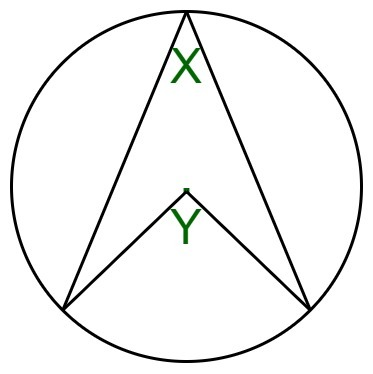# Angle subtended by an arc at the centre of a circle

• Last Updated : 10 Mar, 2022

Given the angle subtended by an arc at the circle circumference X, the task is to find the angle subtended by an arc at the centre of a circle.
For eg in the below given image, you are given angle X and you have to find angle Y.Examples:

Input: X = 30
Output: 60
Input: X = 90
Output: 180

Approach:

• When we draw the radius AD and the chord CB, we get three small triangles.
• The three triangles ABC, ADB and ACD are isosceles as AB, AC and AD are radiuses of the circle.
• So in each of these triangles, the two acute angles (s, t and u) in each are equal.
• From the diagram, we can see

`D = t + u (i)`
• In triangle ABC,

```s + s + A = 180 (angles in triangle)
ie, A = 180 - 2s  (ii)```
• In triangle BCD,

```(t + s) + (s + u) + (u + t) = 180 (angles in triangle again)
so 2s + 2t + 2u = 180
ie 2t + 2u = 180 - 2s (iii)```
`A = 2t + 2u = 2D from (i), (ii)  and (iii)`
• Hence Proved that ‘the angle at the centre is twice the angle at the circumference‘.

Below is the implementation of the above approach:

## C++

 `// C++ implementation of the approach` `#include ``using` `namespace` `std;` `// Function to find Angle``// subtended by an arc``// at the centre of a circle``int` `angle(``int` `n)``{``    ``return` `2 * n;``}` `// Driver code``int` `main()``{``    ``int` `n = 30;``    ``cout << angle(n);` `    ``return` `0;``}`

## Java

 `// Java implementation of the approach``import` `java.io.*;` `class` `GFG``{``    ` `// Function to find Angle subtended``// by an arc at the centre of a circle``static` `int` `angle(``int` `n)``{``    ``return` `2` `* n;``}` `// Driver code``public` `static` `void` `main (String[] args)``{``    ``int` `n = ``30``;``    ``System.out.println(angle(n));``}``}` `// This code is contributed by ajit.`

## Python3

 `# Python3 implementation of the approach` `# Function to find Angle``# subtended by an arc``# at the centre of a circle``def` `angle(n):``    ``return` `2` `*` `n` `# Driver code``n ``=` `30``print``(angle(n))` `# This code is contributed by Mohit Kumar`

## C#

 `// C# implementation of the approach``using` `System;` `class` `GFG``{``    ` `// Function to find Angle subtended``// by an arc at the centre of a circle``static` `int` `angle(``int` `n)``{``    ``return` `2 * n;``}` `// Driver code``public` `static` `void` `Main()``{``    ``int` `n = 30;``    ``Console.Write(angle(n));``}``}` `// This code is contributed by Akanksha_Rai`

## Javascript

 ``

Output:

`60`

Time Complexity: O(1)

Auxiliary Space: O(1)

My Personal Notes arrow_drop_up